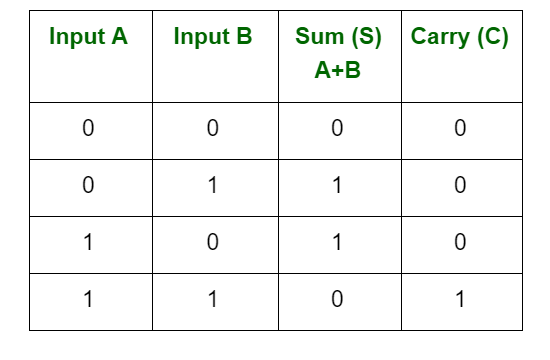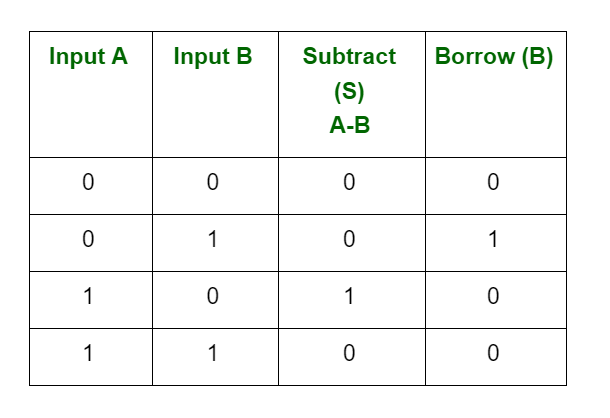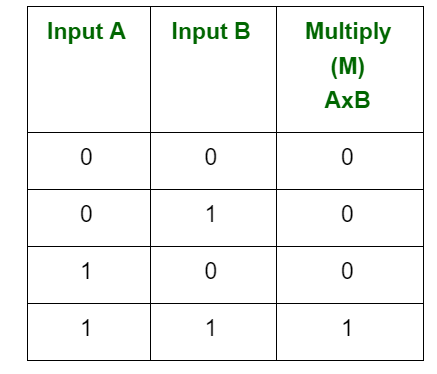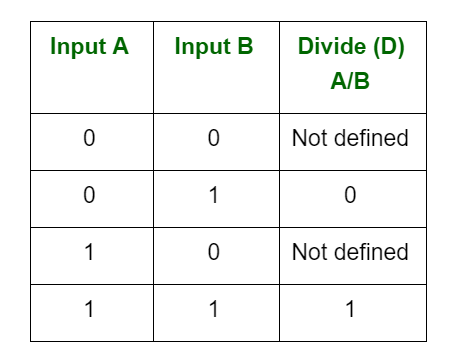# Arithmetic Operations of Binary Numbers

Binary is a base-2 number system that uses two states 0 and 1 to represent a number. We can also call it to be a true state and a false state. A binary number is built the same way as we build the normal decimal number.

Binary arithmetic is an essential part of various digital systems. You can add, subtract, multiply, and divide binary numbers using various methods. These operations are much easier than decimal number arithmetic operations because the binary system has only two digits: 0 and 1.

Binary additions and subtractions are performed as same in decimal additions and subtractions. When we perform binary additions, there will be two outputs: Sum (S) and Carry (C).

1. There are four rules for binary addition:2. There are four rules for binary subtraction:Borrow 1 is required from the next higher order bit to subtract 1 from 0. So, the result became 0.

3. There are four rules for binary multiplication:Multiplication is always 0, whenever at least one input is 0.

4. There are four parts in any division: Dividend, Divisor, quotient, and remainder.The result is always not defined, whenever the divisor is 0.

Attention reader! Don’t stop learning now. Get hold of all the important DSA concepts with the DSA Self Paced Course at a student-friendly price and become industry ready.

My Personal Notes arrow_drop_upIf you like GeeksforGeeks and would like to contribute, you can also write an article using contribute.geeksforgeeks.org or mail your article to contribute@geeksforgeeks.org. See your article appearing on the GeeksforGeeks main page and help other Geeks.

Please Improve this article if you find anything incorrect by clicking on the "Improve Article" button below.